# Bay trimesh¶

## Visualizing water depth into the Chesapeake and Delaware Bays¶

Many natural phenomena exhibit wide differences in the amount they vary across space, with properties varying quickly in some regions of space, and very slowly in others. These differences can make it challenging to make feasible, accurate models using a fixed sampling grid. For instance, for hydrology modelling, areas that are largely flat and uniform can be approximated with just a few samples, while areas of sharp elevation change need many samples per unit area to have a faithful representation of how water will flow. Datashader's support for irregular triangular meshes allows datasets from such simulations to be rendered onscreen efficiently. This notebook shows an example of rendering a dataset from the Chesapeake and Delaware Bay off the US Eastern coast, using data downloaded from the Datashader examples. Once Datashader and the dataset are installed, you can run this notebook yourself to get a live version with interactive zooming for the plots that support it.

First, let's load the data file and take a look at it:

In :
import datashader as ds, datashader.transfer_functions as tf, datashader.utils as du, pandas as pd

fpath = './data/Chesapeake_and_Delaware_Bays.3dm'
names=('row_type', 'cmp1', 'cmp2', 'cmp3', 'val'), index_col=1)

print(len(df))

1626793

Out:

row_type cmp1 cmp2 cmp3 val
1 E3T 29.0 2.0 1.0 3.0
2 E3T 30.0 2.0 29.0 3.0
3 E3T 3.0 2.0 30.0 3.0
4 E3T 31.0 3.0 30.0 3.0
5 E3T 31.0 4.0 3.0 3.0

row_type cmp1 cmp2 cmp3 val
560834 ND -77.101109 38.913535 -0.094789 NaN
560835 ND -77.102765 38.912799 10.298739 NaN
560836 ND -77.103396 38.914439 6.229204 NaN
560837 ND -77.102260 38.915073 -0.423308 NaN
560838 ND -75.964304 36.829522 -6.225454 NaN

Here we have 1.6 million rows of data, some marked 'ND' (vertices defined as lon,lat,elevation) and others marked 'E3T' (triangles specified as indexes into the provided vertices, in order starting with 1 (i.e. like Matlab or Fortran, not typical Python)).

We can extract the separate triangle and vertex arrays we need for Datashader:

In :
e3t = df[df['row_type'] == 'E3T'][['cmp1', 'cmp2', 'cmp3']].values.astype(int) - 1
nd  = df[df['row_type'] == 'ND' ][['cmp1', 'cmp2', 'cmp3']].values.astype(float)
nd[:, 2] *= -1 # Make depth increasing

verts = pd.DataFrame(nd,  columns=['x', 'y', 'z'])
tris  = pd.DataFrame(e3t, columns=['v0', 'v1', 'v2'])

print('vertices:', len(verts), 'triangles:', len(tris))

vertices: 560838 triangles: 1065955


We'll also precompute the combined mesh data structure, which doesn't much matter for this 1-million-triangle mesh, but would save time for plotting for much larger meshes:

In :
%time mesh = du.mesh(verts,tris)

CPU times: user 36 ms, sys: 52.2 ms, total: 88.2 ms
Wall time: 85.9 ms


We can now visualize the average depth at each location covered by this mesh (with darker colors indicating deeper areas (higher z values, since we inverted the z values above)):

In :
cvs = ds.Canvas(plot_height=900, plot_width=900)
%time agg = cvs.trimesh(verts, tris, mesh=mesh)

CPU times: user 2 s, sys: 3.92 ms, total: 2 s
Wall time: 2 s

Out: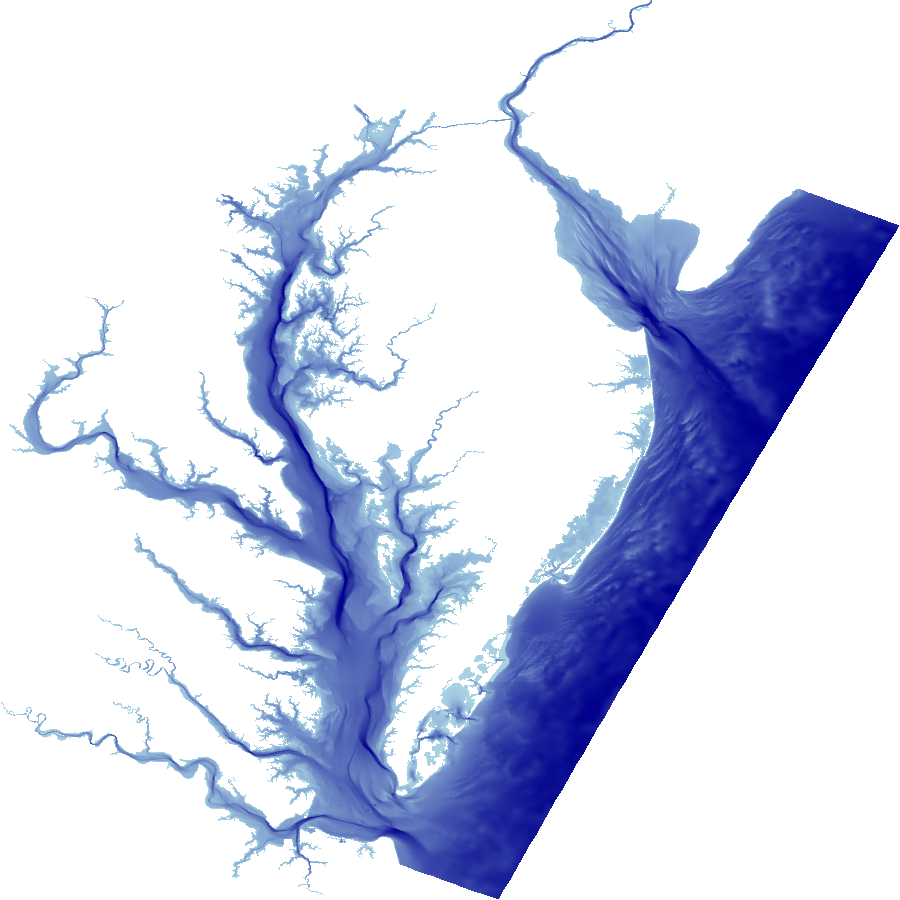When working with irregular grids, it's important to understand and optimize the properties of the mesh, not just the final rendered data. Datashader makes it easy to see these properties using different aggregation functions:

In :
cvs = ds.Canvas(plot_height=400, plot_width=400)

tf.shade(cvs.trimesh(verts, tris, mesh=mesh, agg=ds.count()),   name="count", how='linear'),

Out:
 mean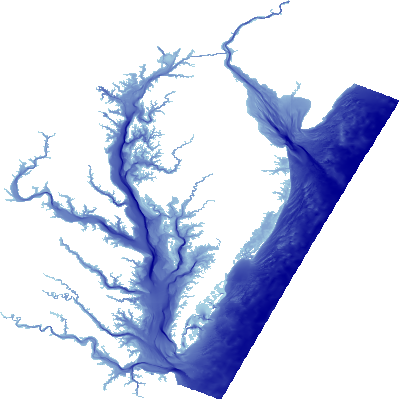any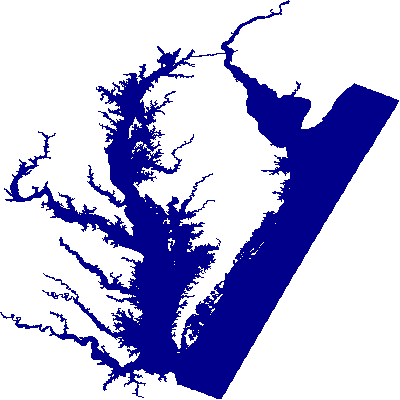count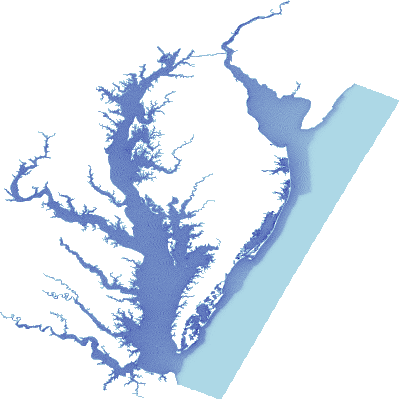std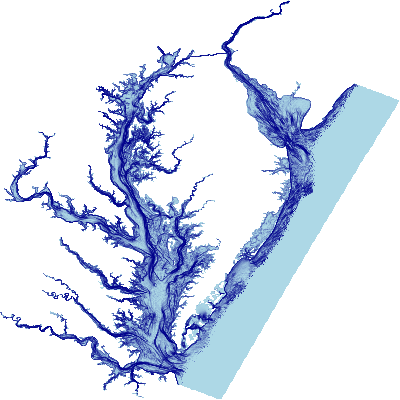Here "any" shows the areas of the plane that are covered by the mesh (for constructing a raster mask), "count" shows how many triangles are used in the calculation of each pixel (with few triangles in the offshore area in this case, and more around the complex inland geometry), and "std" showing how much the data varies in each pixel (highlighting regions of steep change). "max" and "min" can also be useful for finding unexpected areas in the mesh or simulation results, e.g. deep troughs too thin to show up in the plot directly.

Note that before calling tf.shade(), the result of cvs.trimesh is just an xarray array, and so you can run any algorithm you want on the aggregate to do automatic checking or transformation as part of a processing pipeline.

By default, the results are bilinearly interpolated between values at each vertex, but if interpolation is turned off, the average of the values at each vertex is used for the entire triangle:

In :
cvs = ds.Canvas(plot_height=420, plot_width=420, x_range=(-76.56, -76.46), y_range=(38.78, 38.902))
from colorcet import bmy as c

tf.Images(tf.shade(cvs.trimesh(verts, tris, mesh=mesh, interp=True),  cmap=c, name="Interpolated"),
tf.shade(cvs.trimesh(verts, tris, mesh=mesh, interp=False), cmap=c, name="Raw triangles"))

Out:
 Interpolated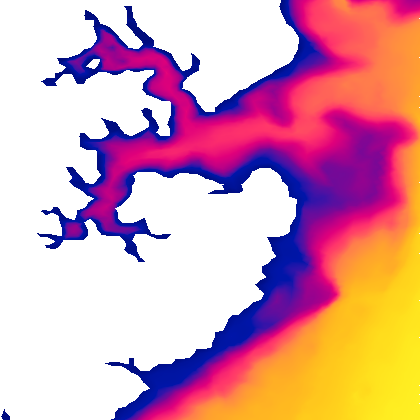Raw triangles